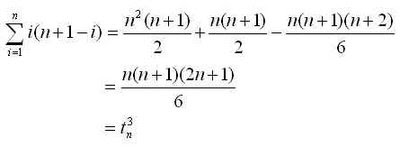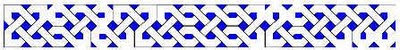## Friday, January 30, 2009

### The Sequence of PrimesAs I make my way through Hardy & Wright's An Introduction to the Theory of Numbers,  I am hoping to work it into my recreational math pursuits - coming up with interesting (but not too heavy) activities that correspond roughly to the material in the text.

The first two chapters are on the sequence of primes. Here's the activity: obtain a list of primes, import them into Fathom, and construct plots that explore pn and pi(n) and other aspects of the sequence that manifest themselves in the first couple of thousand terms.

Here is one of the places you can get your list of primes: http://primes.utm.edu/. In my Fathom experiment, I imported the first 2262 prime numbers.

If you import a sequential list of primes into Fathom (under the attribute prime) and add another attribute n=caseindex, you can create two nice plots. Plot A should have prime as the x axis and n  as the y axis. This shows the function pi(n). To this plot you should add the function = x/ln(x) and visually compare the two curves. Plot B should have the x and y axis reversed. On this graph, plotting the function y = x*ln(x) shows how closely this approximation for pn (the nth prime) comes to the actual values.

You can add further attributes to look at the distance between primes dist=prime-prev(prime), and also the frequency of twin primes is_twin = (dist=2)or(next(dist)=2).

You can also add attributes to keep a running count of twin_primes, and to keep a running average of the twin_primes. The plot above shows how the ratio of tiwn primes diminishes as the number of primes increases. The plot at the top of the post was made by bringing the same data into Tinkerplots - it suggests the distribution of primes and twin primes (in blue) in the numbers up to the 2262nd prime.

## Monday, January 26, 2009

### Triangular Numbers and Euler’s Number Triangle

As mentioned in a few places (such as here, and here), there is a nice identity stating that a square number can be written as the sum of two subsequent triangular numbers.Here we are writing $$t^d_n$$ for the nth triangular of dimension d (d=2 are the flat polygonals, d=3 for they pyramidal polygonals, etc.)

There is also a nice relationship that connects cubes to polygonal numbers. It turns out that a cube of spheres can be unfolded into a packed-hexagonal pyramid. The “packed hexagonals” or “centered hexagonals” are not quite the usual hexagonal numbers – instead these are hexagons of dots with the gaps filled in. The picuture below shows how square numbers fill the gaps of the hexagonals perfectly to form the “packed hexagonals,” and how these in turn can be stacked to form a cube. Here we are using $${ph}^d_n$$ for “packed hexagonals” $$h^d_n$$ for hexagonals, $$s^d_n$$ for squares, and $$t^d_n$$ for triangular numbers.Combining this result with the "triangulation" identities we have:This gives us three nice identities for powers of n:It turns out that these identities generalize for other positive integer powers of n. Every $$n^d$$ can be written as a sum of $$t^d_i$$ where i ranges from $$n$$ to $$n+1-d$$. (for any i less than 1, these terms are zero)
1.Write out the sequence of $$n^d$$ for at least $$2d-2$$ terms. Take the finite difference of this sequence $$d-2$$ times (this reduces the sequence down to “2-dimensional” numbers, allowing us to use the 2-dimensional triangular numbers in our calculations).

2.The first term of the new sequence should be 1. Eliminate the first term by subtracting $$t^2_n$$ from this sequence. This means that our sum begins with $$t^d_n$$, with a coefficient of 1. Ensure that the $$t^2_n$$ values are subtracted from the corresponding terms of the sequence.

3. Now, the sequence has a new first term which is A. Eliminate this term by subtracting A $$t^2_n$$ from the sequence. A is the coefficient of $$t^d_{n-1}$$.

4. Repeat step 3, eliminating the first term of the sequence each time with a multiple of $$t^2_n$$, which provides the coefficient for the next value of $$t^d_i$$.

5.The process ends when all terms in the $$n^d$$ sequence is eliminated, which happens at the dth step.
Carrying out this process for a few more powers of n, we end up with:In general, we seem to have:
where the coefficients A(i,k) have the nice properties:
The coefficients are naturally analogous to the binomial coefficients, and can be arranged in a triangle like Pascal’s.These coefficients are known as Eulerian numbers, and the construction above is known as Euler’s Number Triangle (not to be confused with the geometric construction called the Euler Triangle).

## Thursday, January 22, 2009

### Metaphors and Mathematics 4If mathematics is a game, then playing some game is doing mathematics, and in that case why isn't dancing mathematics too?
Ludwig Wittgenstein - Remarks on the Foundations of Mathematics

Mathematics is often described metaphorically - the  forms that these metaphors take include the organic, mechanical, classical, and post-modern, among countless others. Within these metaphors, mathematics may be a tool, or set of tools, a tree, part of a tree, a vine, a game, or set of games, and mathematicians in turn may be machines, game-players, artists, inventors, or explorers.

Despite the many metaphors used to describe mathematics, in popular discourse mathematics is often reduced to one of its parts, being metonymically described as merely about numbers, formulas, or some other limited aspect. Metaphor is a more complete substitution of ideas than metonymy - allowing us to link concepts that do not appear to have any direct relationship. Perhaps, metaphoric language that elevates and expands our ideas about mathematics is used by enthusiasts to counter the more limited and diminishing metonymic descriptions that are often encountered.

Attempts to describe and elevate mathematics through metaphor seem to fall short, however. Our usual way of thinking about things is to inquire about their meaning - a meaning that is assumed to lie beneath or beyond mere appearances. Metaphor generally relies on making connections between concepts on this deeper level. The sheer formalism of mathematics frustrates this usual way of thinking, and leaves us grasping for a meaning that is constantly evasive. The sheer number and variety of the  many metaphors for mathematics suggests that no single convincing one has yet been found. It may be that the repeated attempts to find such a unifying metaphor represents an ongoing and forever failing attempt to grapple with the purely formal character of mathematics; and it may be that the formal nature of mathematics will always shake off any metaphor that attempts to tie it down.

## Friday, January 16, 2009

### The Humble Multiplication Table, 1A surprising relationship found in the multiplication table is that the sum of the entries in the main upwards diagonal and the diagonal above it is equal to the sum of the entries in the main downwards diagonal. What is also surprising is that this is but one among several observations about the multiplication table that can be expressed in terms of polygonal numbers.

This relationship involves three-dimensional triangular numbers (triangle-based pyramidal numbers, or tetrahedral numbers), and three-dimensional square numbers (square-based pyramidal numbers). Some values for these, and a few other polygonals, are shown below.To see why this relationship holds, first note that the sum of the entries in the nth upward diagonal in the multiplication table is equal to the nth three-dimensional triangular number.Second, observe that he entries in the main down diagonal are square numbers (two-dimensional), so the sum of the main down diagonal is the nth three-dimensional square number.

Finally, we use the fact that a square number (of any dimension) can be split into two triangular numbers (of the same dimension), which gives us the surprising result above.

## Wednesday, January 14, 2009

### Invention / DiscoveryI have been doing mathematics for 57 years. What is my experience? Do I discover or invent? Am I a James Cook, finding what was already there, or a Thomas Edison, bringing something new into being?… My answer is unequivocal: for me, the experience is one of invention.
Edward Nelson, (2002) Syntax and Semantics

Is mathematics invention or discovery? When mathematicians come upon their results are they just producing elaborate mental constructions which have no actual reality… or are mathematicians really uncovering truths which are, in fact, already ‘there’ – truths whose existence is quite independent of the mathematicians’ activities? I think that, by now, it must be quite clear to the reader that I am an adherent of the second, rather than the first view…
Roger Penrose, (1989) The Emperor’s New Mind. Oxford University Press, p126

The mathematician is an inventor, not a discoverer.
Ludwig Wittgenstein, (1938) Remarks on the Foundations of Mathematics, I-168